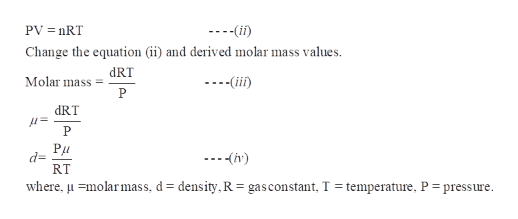# In the gas phase, nitrogen dioxide is actually a mixture of nitrogen dioxide (NO2) and dinitrogen tetroxide (N2O4). If the density of such a mixture is 3.06 g/L at 74°C and 1.70 atm,calculate the partial pressures of the gases and KP for the dissociation of N2O4.Pressure of NO2: ?atm Pressure of N2O4: ?atm KP=?

Question
104 views

In the gas phase, nitrogen dioxide is actually a mixture of nitrogen dioxide (NO2) and dinitrogen tetroxide (N2O4). If the density of such a mixture is 3.06 g/L at 74°C and 1.70 atm,calculate the partial pressures of the gases and KP for the dissociation of N2O4.

Pressure of NO2: ?atm

Pressure of N2O4: ?atm

KP=?

check_circle

Step 1

The equilibrium reaction of the nitrogen dioxide N2O4 -

The given equilibrium reaction has a homogeneous process ie., dissociation reaction and formation reaction, then their equilibrium constant is given by Kp, partial pressure.

Step 2

Given,

Density = 3.06 g/L

Temperature = 74°C

Pressure = 1.70 atm

Now we have to calculate the partial pressure of all the gases and the Kp value for the dissociation of N2O5.

Step 3

According to an ideal...help_outlineImage TranscriptionclosePV nRT (i) Change the equation (ii) and derived molar mass values. dRT Molar mass (ii) P dRT Р Pu d= RT (h) where, umolarmass, d = density.,R = gasconstant, T = temperature, P = pressure fullscreen

### Want to see the full answer?

See Solution

#### Want to see this answer and more?

Solutions are written by subject experts who are available 24/7. Questions are typically answered within 1 hour.*

See Solution
*Response times may vary by subject and question.
Tagged in

### Chemistry